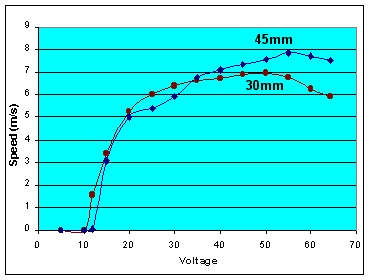Mark II Results - Voltage

What is the relationship between muzzle speed versus capacitor voltage? And how does the energy conversion efficiency relate to voltage?

Capacitor Voltage

I measured speed at various applied voltages, and plotted the results below. The test was repeated with another projectile which was 50% longer than the first. Using significantly different lengths will tell us more about this dependence.Volts
30mm
Speed
45mm
Speed
5v  0  0
10v  0  0
12v 1.56 m/s  0.07 m/s
15v 3.40 3.06
20v 5.24 5.00
25v 6.01 5.42
30v 6.40 5.90
35v 6.61 6.80
40v 6.74 7.09
45v 6.91 7.34
50v 6.96 7.60
55v 6.75 7.85
60v 6.26 7.71
64v 5.91 7.51

A minimum voltage of 10v was required to make the coilgun work at all. At low voltages (10 to 30v) the speed is extremely sensitive to voltage. The shape of the curve suggests a parabola, i.e. Speed = K*SQRT(volts - 10). At medium voltages (30 to 60v) the speed is fairly flat, and varies about 10% with respect to voltage.

At higher voltages (60+ volts) the speed drops off. The gun sounded a little distressed, as it made definite thumping noises during firing. I believe this is because at higher speeds the projectile is trying to leave the coil before the magnetic field has died, and it decelerates before it is gone.

There is a curious dip in the 45mm curve from 20v to 30v. I have no explanation for it. It would appear to be a measurement error, but I repeated the testing over this range and got the same results.

The longer projectile went 12% faster than the shorter one. This suggests an even longer projectile may go a little faster yet. The effect is not large; notice that one projectile was 50% longer than the other.

Conclusions - A key lesson is how to tune the coilgun with voltage. Notice the sharp knee around 20 to 30 volts. Then only 10% gain is achieved when the voltage is doubled to 60 volts. A reasonable tuning strategy is to gradually increase the voltage until this knee is identified. Further voltage increases will stress the circuitry without providing significant benefits.

Energy Conversion Efficiency

Out of curiosity, I also calculated the machine's efficiency at each voltage, as a ratio of kinetic energy (1/2 m v2) to stored energy (1/2 C V2).

Volts
30mm
Efficiency
45mm
Efficiency
12v 0.5%  0.0%
15v 1.5% 1.8%
20v 2.0% 2.7%
25v 1.7% 2.0%
30v 1.3% 1.7%
35v 1.1% 1.6%
40v 0.8% 1.3%
45v 0.7% 1.1%
50v 0.6% 1.0%
55v 0.4% 0.9%
60v 0.3% 0.7%
64v 0.2% 0.6%

This model is up to 2.7% efficient in converting stored energy into kinetic energy. Frankly, this is rather higher than I expected.

The capacitors store 4.8 joules at 20vdc. The 31.5mm projectile weighs 7.07 grams, and the 44.7mm projectile weighs 10.07 grams. The kinetic energy is computed from E = 0.5 m v^2, so the approximate energy at 5.24 m/s is 0.0971 joules. The energy conversion efficiency is Eout/Ein.

Conclusions - The important point here is to use the efficiency figure to design the next coilgun. Assuming the next model (or a higher voltage) is this efficient, you can work backwards to estimate the exit speed. From the speed, you can estimate the timing needed for the coil. And from the timing, you can compute the necessary inductance for a given storage capacitor. Neat, eh?!

 < Previous Page 18 of 18 Next >